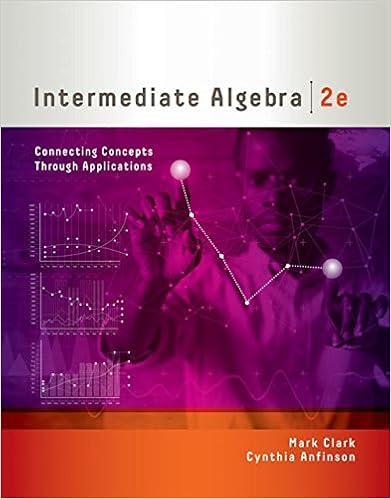# The base of a solid sitting on the xy plane is the

• Test Prep
• 9
• 100% (2) 2 out of 2 people found this document helpful

This preview shows page 4 - 8 out of 9 pages.

##### We have textbook solutions for you!
The document you are viewing contains questions related to this textbook.The document you are viewing contains questions related to this textbook.
Chapter 4 / Exercise 46
Intermediate Algebra: Connecting Concepts through Applications
ClarkExpert Verified
6. The base of a solid sitting on thexy-plane is the region enclosed by the curvesx=-π4,y= 2 sinxandy= 2 cosxover the interval-π4xπ4.Suppose that each cross section of the solidperpendicular to thex-axis is an equilateral triangle (i.e.all sides have equal length).Find thevolume of the solid.xy3π33π53π73π93π(a)2(b)2(c)2(d)2(e)2
7. The region enclosed by the graph of the functiony= 2x2and the liney= 16 is rotated about they-axis to generate a solid of revolution. Find the average value of the cross section areas of the solidwhich are perpendicular to they-axis.
8. Letfbe a positive differentiable function satisfyingf(0) = 1,f(1) = 2,f(0) = 2,f(1) = 3.Evaluate the integralintegraldisplay10f(x) ln(f(x))dx.
##### We have textbook solutions for you!
The document you are viewing contains questions related to this textbook.The document you are viewing contains questions related to this textbook.
Chapter 4 / Exercise 46
Intermediate Algebra: Connecting Concepts through Applications
ClarkExpert Verified
49. The graph of the functiony=x+ 2, where 0x10, is rotated about thex-axis to generate asurface of revolution. Find the area of the surface.
10. Find the slope of the tangent line to the curve defined by the polar equationr= 3 + sin 2θ-cos2θat the point on the curve with angular coordinateθ=π.xy(a)12(b)23(c)1(d)34(e)45
11. A curve in thexy-plane is defined by the polar equationr=sin 2θ, whereπ8θπ4. Which ofthe following definite integrals is the arc length of the curve?radicalbigg
8
5Part II: Answer each of the following questions.12. [15 pts] The region enclosed by the curvesy= ln(1 +x2) andy= ln 17 is rotated about they-axisto generate a solid of revolution.(a) Express the volume of the solid as a definite integral.[5 pts]
(b) Find the volume of the solid.[10 pts]
613. [15 pts]A container has the shape of a bowl obtained by rotating the curvey= 2x2,-3x3,around they-axis.The lowest point of the container is 3 m above the ground.Find the work
•••